RRB JE ME (CBT I) Mock Test- 3

# RRB JE ME (CBT I) Mock Test- 3

Test Description

## 100 Questions MCQ Test RRB JE Mock Test Series for Mechanical Engineering (ME) | RRB JE ME (CBT I) Mock Test- 3

RRB JE ME (CBT I) Mock Test- 3 for Railways 2023 is part of RRB JE Mock Test Series for Mechanical Engineering (ME) preparation. The RRB JE ME (CBT I) Mock Test- 3 questions and answers have been prepared according to the Railways exam syllabus.The RRB JE ME (CBT I) Mock Test- 3 MCQs are made for Railways 2023 Exam. Find important definitions, questions, notes, meanings, examples, exercises, MCQs and online tests for RRB JE ME (CBT I) Mock Test- 3 below.
Solutions of RRB JE ME (CBT I) Mock Test- 3 questions in English are available as part of our RRB JE Mock Test Series for Mechanical Engineering (ME) for Railways & RRB JE ME (CBT I) Mock Test- 3 solutions in Hindi for RRB JE Mock Test Series for Mechanical Engineering (ME) course. Download more important topics, notes, lectures and mock test series for Railways Exam by signing up for free. Attempt RRB JE ME (CBT I) Mock Test- 3 | 100 questions in 90 minutes | Mock test for Railways preparation | Free important questions MCQ to study RRB JE Mock Test Series for Mechanical Engineering (ME) for Railways Exam | Download free PDF with solutions
 1 Crore+ students have signed up on EduRev. Have you?
RRB JE ME (CBT I) Mock Test- 3 - Question 1

### If length, breadth, and height of a cuboid is increased by a%, b%, and c% respectively, then its volume is increased by-

Detailed Solution for RRB JE ME (CBT I) Mock Test- 3 - Question 1 Let us suppose that each side of the cuboid be 100 units. Then its volume 1003 units

Now new sides of the cuboid are: (100 + a), (100 + b) and (100 + c)

Then its new volume = (100 + a)(100 + b)(100 + c)

= 1003+1002(a+b+c)+100(ab+bc+ca)+abc

Then % change in volume

= 1002(a+b+c)+100(ab+bc+ca)+abc/1003) × 100

= (a+b+c + ab+bc+ca/100 + abc/(100)2)%

RRB JE ME (CBT I) Mock Test- 3 - Question 2

### Surendra started a mocktail (soft drink + soda) counter. Initially, he had 140 liters of mocktail, which had 30% soda in it. He sold 20 liters of the mocktail. Then he added an equal amount of soft drink and soda. Now the ratio of soda to soft drink became 2:3. How much soda was added later on?

Detailed Solution for RRB JE ME (CBT I) Mock Test- 3 - Question 2 Initial amount of soft drink = 100% mock tail – 30% soda = 70% soft drink

Surendra sold 20 liter mock tail, so now he has 140 - 20 = 120 liters mocktail

In this 120l mock tail, the ratio will be the same, i.e., 30% soda and 70% soft drink.

So, amount of soft drink = 70% of 120 = 84 liters

So, Amount of soda = 120 – 84 = 36 liters

Now, let Surendra add x liters of soft drink and x liters of soda

36+x/84+x = 23

108 + 3x = 168 + 2x

x = 60 liters = amount of soda added

RRB JE ME (CBT I) Mock Test- 3 - Question 3

### Find the remainder in the expression 557×653×672/9.

Detailed Solution for RRB JE ME (CBT I) Mock Test- 3 - Question 3

557×653×672/9

557 is divided by 9, remainder = 8

653 is divided by 9, remainder = 5

672 is divided by 9, remainder = 6

So, now multiplication of remainders 8×5×6 = 240 is divided by 9, find the required remainder.

Required remainder = 6

RRB JE ME (CBT I) Mock Test- 3 - Question 4

The bar-graph given above shows the percentage of marks obtained by six students namely P, Q, R, S, T and U in a class test. There were three class tests viz. Hindi, English and Science and each test was of 20 marks. Any student scoring less than 58% marks is considered failed in the exams.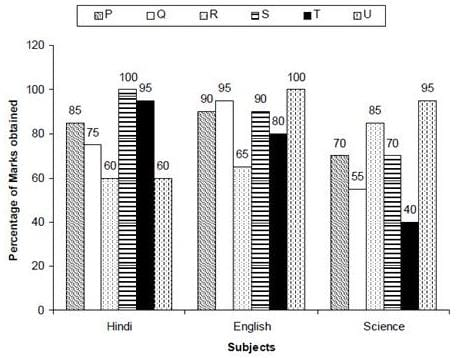By what percentage, the marks scored by P in Hindi more or less than that scored by T in Science?

Detailed Solution for RRB JE ME (CBT I) Mock Test- 3 - Question 4

Marks scored by P in Hindi = 20 × 0.85 = 17

Marks scored by T in science = 20 × 0.40 = 8

Percentage value

= 17−8/8 × 100 = 112.5%

RRB JE ME (CBT I) Mock Test- 3 - Question 5

Two poles of the height 12 m and 17 m stand vertically upright on a plane ground. If the distance between their feet is 12 m, find the distance between their tops.

Detailed Solution for RRB JE ME (CBT I) Mock Test- 3 - Question 5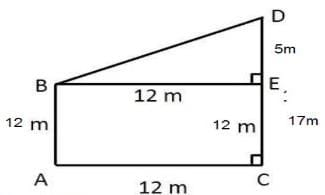In triangle BDE,

BD2 = BE2 + DE2

= 52 + 122 = 144 + 25 = 169

BD = 13 m

RRB JE ME (CBT I) Mock Test- 3 - Question 6

What is the difference between CI and SI, if the sum is Rs. 8,000 for 4 years at a rate of 4%?

Detailed Solution for RRB JE ME (CBT I) Mock Test- 3 - Question 6

Difference between SI and CI = Sum × r2 × (300+r)/1003

= 8000 × 42 × (300+4)/1003

= 38912000/1000000 = 38.91

RRB JE ME (CBT I) Mock Test- 3 - Question 7

One glass has wine and soda in the ratio 4:3 while other same quantity of glass has in the ratio 3:2. If both glasses are poured in a vessel, what will be the final ratio of soda to wine in the vessel?

Detailed Solution for RRB JE ME (CBT I) Mock Test- 3 - Question 7

Glass 1 : Wine : soda = 4 : 3

Glass 2 : Wine : soda = 3 : 2

Vessel Wine = Glass 1 + Glass 2 = 4/7+3/5 = 41/35

Vessel soda = Glass 1 + Glass 2 = 3/7+2/5 = 29/35

Soda to wine ratio = 29/35 : 41/35 = 29 : 41

RRB JE ME (CBT I) Mock Test- 3 - Question 8

3 different products (in Thousands) produced by a company in five different years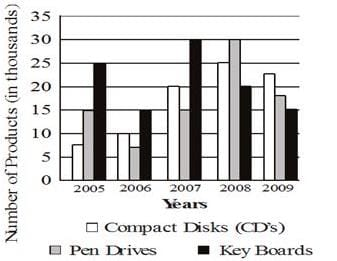What was the total number of all the products produced by the company in the year 2005 and 2007 together?

Detailed Solution for RRB JE ME (CBT I) Mock Test- 3 - Question 8 Required number of all products = (7.5+15+25+20+15+30) thousand

= 112500

RRB JE ME (CBT I) Mock Test- 3 - Question 9

The bar-graph given above shows the percentage of marks obtained by six students namely P, Q, R, S, T and U in a class test. There were three class tests viz. Hindi, English and Science and each test were of 20 marks. Any student scoring less than 58% marks is considered failed in the exams.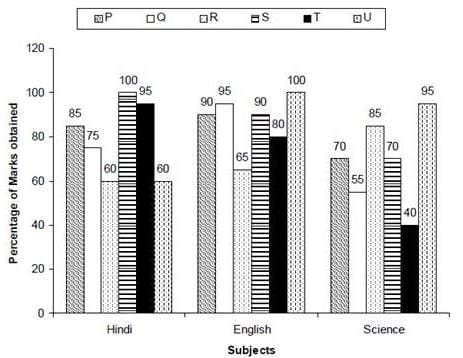What is the average score of the class in these three tests?

Detailed Solution for RRB JE ME (CBT I) Mock Test- 3 - Question 9 As we don't know the total number of student in the class. Hence, we cannot determine the average of marks of the class.
RRB JE ME (CBT I) Mock Test- 3 - Question 10

Three-fourth part of a tank is filled with water. 50% of the water is removed from the tank and 60 liters of pure milk is added to it. If now the ratio of milk-water in the tank becomes 5:4, then the capacity of the tank will be:

Detailed Solution for RRB JE ME (CBT I) Mock Test- 3 - Question 10

As the new ratio of milk and water is 5:4

The quantity of milk = 60 liters and hence the quantity of water has to be 48 liters.

Now, 1/2 of 3/4th of the capacity of tank = 48 liters

So, capacity of the tank = 48×2×4/3 = 128 liters

RRB JE ME (CBT I) Mock Test- 3 - Question 11

Seats for Science, Arts and Commerce in a school are in the ratio 6:9:10. There is a proposal to increase these seats by 30%, 20% and 65% respectively. What will be the ratio of increased seats?

Detailed Solution for RRB JE ME (CBT I) Mock Test- 3 - Question 11 Originally, let the number of seats for Science, Arts and Commerce be 6x, 9x and 10x respectively.

Number of increased seats are (130% of 6x), (120% of 9x) and (165% of 10x).

(130/100 × 6x) : (120/100 × 9x) : (165/100×10x)

= 78x : 108x : 165x

= 26 : 36 : 55

RRB JE ME (CBT I) Mock Test- 3 - Question 12

50% of a class of 120 students passed in physics and only 20% not passed in chemistry. The maximum possible number of students who can neither passed in physics nor in chemistry is?

Detailed Solution for RRB JE ME (CBT I) Mock Test- 3 - Question 12 Number of students does not pass in physics = 50% of 120 = 60

Number of students does not pass in chemistry = 20% of 120

= 20/100×120 = 24

So, the maximum possible number of students who can neither passed in physics nor in chemistry is = 24

RRB JE ME (CBT I) Mock Test- 3 - Question 13

What is the positive square root of

Detailed Solution for RRB JE ME (CBT I) Mock Test- 3 - Question 13 [19 + 4√21]

= (√7)2 + (2√3)2 + 2 x 2√3 x √7

Which is a perfect square,

= (√7 + 2√3)2

Its square root = √7 + 2√3

RRB JE ME (CBT I) Mock Test- 3 - Question 14

Three different companies of bikes used by persons (in lacs)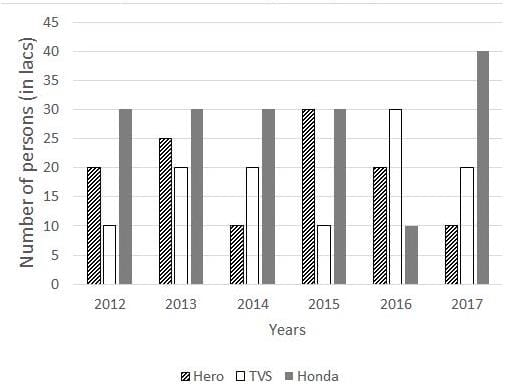Find the ratio of average of hero bikes used in the 2012 and 2017 and average of Honda bikes used in the same years.

Detailed Solution for RRB JE ME (CBT I) Mock Test- 3 - Question 14 Average of hero bikes used in 2012 and 2017 = 2000000+1000000/2 = 1500000

Average of honda bikes using in 2012 and 2017 = 3000000+4000000/2 = 3500000

Required ratio = 1500000/3500000 = 3 : 7

RRB JE ME (CBT I) Mock Test- 3 - Question 15

Three different companies of bikes used by persons (in lacs)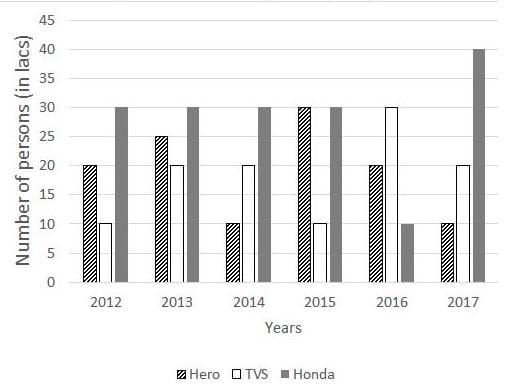Find out the percentage increase or decrease in the number of persons using Hero from 2014 to 2015?

Detailed Solution for RRB JE ME (CBT I) Mock Test- 3 - Question 15 Number of persons using Hero bikes in 2014 = 1000000

Number of persons using Hero bikes in 2014 = 3000000

Required percentage increase = 3000000−1000000/1000000×100

= 2000000/1000000×100 = 200%

= 200 %

RRB JE ME (CBT I) Mock Test- 3 - Question 16

Amit and Bhanu invest in a business in the ratio 3 : 2. If 5% of the total profit goes to charity and Amit's share is Rs. 8550, then find the profit share of Bhanu.

Detailed Solution for RRB JE ME (CBT I) Mock Test- 3 - Question 16 Let the total profit be Rs. 100.

After paying to charity, Amit's share = Rs. (95× 3/5 ) = Rs. 57

If Amit’s share is Rs. 57, total profit = Rs. 100

If Amit's share is Rs. 8550 then total profit = 8550 × 100/5

= 15000

Bhanu’s share = (15000×95/100×25) = Rs. 5700

RRB JE ME (CBT I) Mock Test- 3 - Question 17

In triangle PQR, A is the point of intersection of all the altitudes and B is the point of intersection of all the angle bisectors of the triangle. If ∠PBR = 1050, then what is the value of ∠PAR?

Detailed Solution for RRB JE ME (CBT I) Mock Test- 3 - Question 17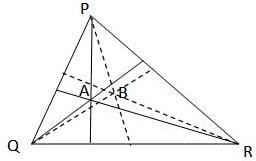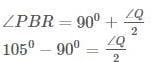∠PQR = 300

∠PAR = 1800 - 300 = 1500

RRB JE ME (CBT I) Mock Test- 3 - Question 18

The average monthly income of A and B is Rs 7760. The average monthly income of B and C is Rs. 10990 and that of C and A is Rs. 9070. What is the annual income of B?

Detailed Solution for RRB JE ME (CBT I) Mock Test- 3 - Question 18

Total income of A and B = 2 x 7760

So, A+B = 15520 ... (i)

Similarly,

B +C = 10990 x 2 = 21980... (ii)

and A+C = 9070 x 2 = 18140 ... (iii)

Adding Eqs. (i), (ii) and (iii),

2(A + B + C) = 55640

⇒ A+B + C =27820 ... (iv)

Subtracting Eq. (iii) from Eq. (iv),

B = 27820 – 18140 = 9680

Hence, annual income of B = 9680 x 12 = Rs. 116160

RRB JE ME (CBT I) Mock Test- 3 - Question 19

If a shopkeeper sells a TV at a discount of 42%, he loses 13%. At what discount should he sell the TV to earn a profit of 5%?

Detailed Solution for RRB JE ME (CBT I) Mock Test- 3 - Question 19

SP = (1−42/100) MP= (1−13/100) CP

MP = 0.87×CP/0.58= 1.5×CP

To earn profit of 5%, let the discount be D%.

New SP= (1+5/100) CP = (1−D/100) MP

1.05CP = (1−D/100) × 1.5 × CP

D= 30%

Short trick:

100 − D1/100 − D2 = 100+P1/100+P2

58/100−D2 = 87/105

⇒ D2 = 100−70 = 30%

RRB JE ME (CBT I) Mock Test- 3 - Question 20

The profit obtained by selling an article for Rs. 856 is twice the loss incurred when the article is sold for Rs. 760. The cost price of the article is?

Detailed Solution for RRB JE ME (CBT I) Mock Test- 3 - Question 20 Let ‘C’ be the cost price of the article.

⇒ (856 - C) = 2(C - 760)

⇒ 3C = 856 + 1520

⇒ 3C = 2376

⇒ C = 792

∴ The cost price of the article = 792

RRB JE ME (CBT I) Mock Test- 3 - Question 21

The length of two parallel chords of a circle of radius 5cm are 6 cm and 8 cm in the same side of the centre. The distance between them is-

Detailed Solution for RRB JE ME (CBT I) Mock Test- 3 - Question 21 Here, O is the centre of the circle of radius 5 cm and AB and CD are two chord of length 8 cm and 6 cm respectively.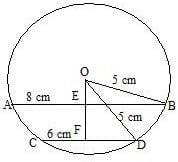∵ Perpendicular drawn from the centre of a circle to the chord bisects the chord.

∴ EB = 4 cm and FD = 3 cm

Now, In ΔΔ OEB

OB2 = OE2 + EB2

RRB JE ME (CBT I) Mock Test- 3 - Question 22

P and Q are standing at a distance of 350 m from each other on a straight road such that P is to the west of Q. Both of them start walking simultaneously towards each other and cover a distance of 50 m each. Thereafter, P turns to his left and walks 290 m while Q turns to his right and walks 80 m. At the end both of them turn to their left such thatP covers a distance of 50 m while Q covers a distance of 100 m. How far and in which direction is Q from P?

Detailed Solution for RRB JE ME (CBT I) Mock Test- 3 - Question 22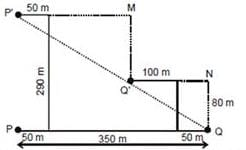Shortest Distance between P and Q = P'Q' =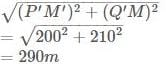Hence Q is 290m from P and in the South- East Direction.

RRB JE ME (CBT I) Mock Test- 3 - Question 23

What percentages of numbers from 1 to 80 have 1 or 9 in the unit's digit?

Detailed Solution for RRB JE ME (CBT I) Mock Test- 3 - Question 23 Numbers from 1 to 80 have 1 or 9 in the unit's digit

1, 9, 11, 19, 21, 29, 31, 39, 41, 49, 51, 59, 61, 69, 71, 79

Number of such number = 16

Required percentage = (16/80 × 100) = 20%

RRB JE ME (CBT I) Mock Test- 3 - Question 24

If tan θ = p/q, then the value of p cos 2θ + qsin 2θ is:

Detailed Solution for RRB JE ME (CBT I) Mock Test- 3 - Question 24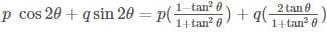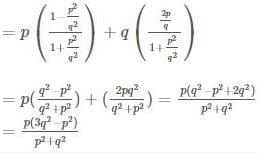RRB JE ME (CBT I) Mock Test- 3 - Question 25

The median of six numbers 6, 7, x – 5, x – 3, 17 and 19, in ascending order, is 11. The value of x is:

Detailed Solution for RRB JE ME (CBT I) Mock Test- 3 - Question 25 The median of six number

= (x−5) + (x−3)/2

= 2x − 8/2

= x − 4

According to Question-

x - 4 = 11

x = 15

RRB JE ME (CBT I) Mock Test- 3 - Question 26

If tan2 θ + cot2 θ = x , what is the value of 1/cosθ.sinθ = ?

Detailed Solution for RRB JE ME (CBT I) Mock Test- 3 - Question 26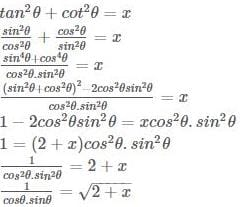RRB JE ME (CBT I) Mock Test- 3 - Question 27

The product of two numbers is 108 and the sum of their squares is 625. The sum of the number is:

Detailed Solution for RRB JE ME (CBT I) Mock Test- 3 - Question 27 Let the Numbers be P and Q.

PQ= 108, P2+Q2 = 625

(P+Q)2 = P2 + Q2 + 2PQ

(P+Q)2 = 625 + 2 × 108

(P+Q)2 = 841

P+Q = 29

RRB JE ME (CBT I) Mock Test- 3 - Question 28

Ria was one more than eleven times as old as Sandhya in January, 2000 and seven more than three times as old as her in January, 2009. How old was Ria in January, 2000?

Detailed Solution for RRB JE ME (CBT I) Mock Test- 3 - Question 28 Let Ria’s and Sandhya’s age in January 2000 be X years and Y years resp.

According to Question-

X = 1+11Y---------- (1)

In January 2009, Ria’s age was (X+9) years and Sandhya’s was (Y+9) years.

So,

X+9 = 7+3(Y+9)

X = 25+3Y------------(2)

On Solving(1) and (2)

X = 34 and Y = 3

Hence. In January 2000 Ria was 34 Years old.

RRB JE ME (CBT I) Mock Test- 3 - Question 29

What is the unit digit in (795 − 357)?

Detailed Solution for RRB JE ME (CBT I) Mock Test- 3 - Question 29

Unit digit in 795 = Unit digit in [(74)23 × 73]

= Unit digit in [(Unit digit in (2401))23 × (343)]

= Unit digit in (123 × 343)

= Unit digit in (343)

= 3

Unit digit in 357 = Unit digit in

=[(34)14 × 31] = Unit digit in [Unit digit in (81)14 × 3]

= Unit digit in [(1)14 × 3]

= Unit digit in (1 x 3)

= Unit digit in (3)

= 3

Unit digit in (795 − 357) = Unit digit in (343 - 3) = Unit digit in (340) = 0.

RRB JE ME (CBT I) Mock Test- 3 - Question 30

A sum of Rs. 4000 becomes Rs. 5800 in 3 years, when invested in a scheme of simple interest. If the same sum is invested in a scheme of compound interest with same yearly interest rate (compounding of interest is done yearly), then what will be the amount (in Rs) after 2 years?

Detailed Solution for RRB JE ME (CBT I) Mock Test- 3 - Question 30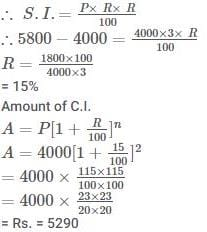RRB JE ME (CBT I) Mock Test- 3 - Question 31

Below are given letters A to Z. Under each capital letter, a small letter is written which is to be used as a code for the Capital Letters: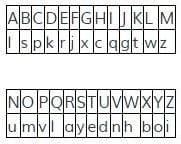In each of the following questions, a group of six Capital Letters is given and its code equivalent is given below. Select the response containing the correct code.

Find the code for 'CHWCLS'

Detailed Solution for RRB JE ME (CBT I) Mock Test- 3 - Question 31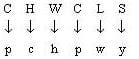RRB JE ME (CBT I) Mock Test- 3 - Question 32

DIRECTIONS: Identify the diagram that best represents the relationship among Iron, Lead, Nitrogen.

Choose the correct venn diagram.

Detailed Solution for RRB JE ME (CBT I) Mock Test- 3 - Question 32 There is no relation between iron, lead and nitrogen.

So,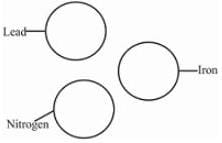RRB JE ME (CBT I) Mock Test- 3 - Question 33

The last day of a century cannot be either

I. Tuesday.

II. Thursday.

III. Saturday.

IV. Sunday.

Detailed Solution for RRB JE ME (CBT I) Mock Test- 3 - Question 33 The last day of century cannot be either Tuesday, Thursday or Saturday.
RRB JE ME (CBT I) Mock Test- 3 - Question 34

Choose the odd one out from the given alternatives.

Detailed Solution for RRB JE ME (CBT I) Mock Test- 3 - Question 34 All except Cabbage grow under-ground.
RRB JE ME (CBT I) Mock Test- 3 - Question 35

DIRECTIONS: In each of the following questions, choose the set of numbers out of the four options that is similar to the given set :(17, 35, 71)

Detailed Solution for RRB JE ME (CBT I) Mock Test- 3 - Question 35 Double the first number + 1 = second number and double the second number + 1 = third number.
RRB JE ME (CBT I) Mock Test- 3 - Question 36

From the given responses, find the missing number in the series.

YW, US, ?, MK

Detailed Solution for RRB JE ME (CBT I) Mock Test- 3 - Question 36 The pattern is as follows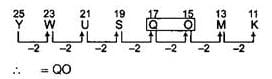RRB JE ME (CBT I) Mock Test- 3 - Question 37

Complete the given series:

6, 12, 30, 56, ?

Detailed Solution for RRB JE ME (CBT I) Mock Test- 3 - Question 37 The given series follows the pattern

(prime number)2 + (prime number)

So, 22+2=4+2=6,

32+3=9+3=12,

52+5=25+5=30,

72+7=49+7=56,

112+11=121+11=132.

Hence the required term will be 132.

RRB JE ME (CBT I) Mock Test- 3 - Question 38

Ram is facing North-West. He turns in clockwise direction by 900, then 1800 in the anti-clockwise direction and 900 in the same direction. Which direction is he facing now?

Detailed Solution for RRB JE ME (CBT I) Mock Test- 3 - Question 38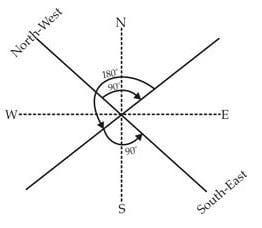He is facing in South-East direction.

RRB JE ME (CBT I) Mock Test- 3 - Question 39

If A means ‘+, ‘B means’-’ C means ‘x’ and D means ÷, then

18 C 14 A 6 B 16 D 4?

Detailed Solution for RRB JE ME (CBT I) Mock Test- 3 - Question 39 According to the question,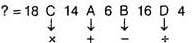= 18 x 14 + 6 - 16 ÷ 4 (using BODMAS rule)

= 18 x 14 + 6 - 4 = 252 + 6 - 4 = 258 - 4 = 254

RRB JE ME (CBT I) Mock Test- 3 - Question 40

Four of the following five pairs have the same relation between their elements and form a group. Which one does not belong to that group?

Detailed Solution for RRB JE ME (CBT I) Mock Test- 3 - Question 40 Only the tiger and the tigress hold the male and female relationship, while the remaining hold the parent and offspring relationship.
RRB JE ME (CBT I) Mock Test- 3 - Question 41

What should come at the place of question mark?

Q1F, S2E, U6D, W21C, ?

Detailed Solution for RRB JE ME (CBT I) Mock Test- 3 - Question 41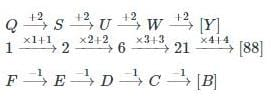RRB JE ME (CBT I) Mock Test- 3 - Question 42

What should come at the place of the question mark?

N5V, K7T, ?, E14P, B19N

Detailed Solution for RRB JE ME (CBT I) Mock Test- 3 - Question 42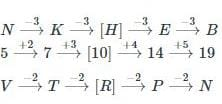RRB JE ME (CBT I) Mock Test- 3 - Question 43

In questions given below select the related letters from the given alternatives.

NLO:RPS::VTW:?

Detailed Solution for RRB JE ME (CBT I) Mock Test- 3 - Question 43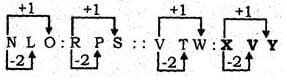RRB JE ME (CBT I) Mock Test- 3 - Question 44

A piece of paper is folded and cut as shown below in the questions figure. From the given answer figures, indicate how it will appear when opened?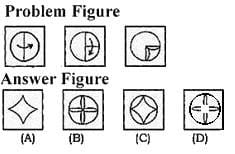Detailed Solution for RRB JE ME (CBT I) Mock Test- 3 - Question 44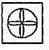RRB JE ME (CBT I) Mock Test- 3 - Question 45

Select the answer figure in which the questions figure is embedded.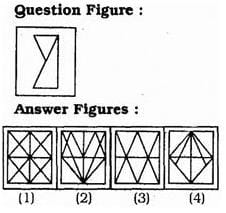Detailed Solution for RRB JE ME (CBT I) Mock Test- 3 - Question 45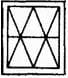RRB JE ME (CBT I) Mock Test- 3 - Question 46

Identify the diagram that best represents the relationship among Profit, Dividend and Bonus.

Detailed Solution for RRB JE ME (CBT I) Mock Test- 3 - Question 46 Bonus and Dividends are different from each other. But both these are parts of profit.
RRB JE ME (CBT I) Mock Test- 3 - Question 47

Four kids P, Q, R and S are up on the ladder. P is further up the ladder than Q, Q is between P and R. If S is further up than P, who is the third from the bottom?

Detailed Solution for RRB JE ME (CBT I) Mock Test- 3 - Question 47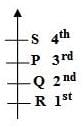P is third from bottom.

RRB JE ME (CBT I) Mock Test- 3 - Question 48

In the following questions, a word is followed by five other words, one of which cannot be formed by using the letters of the given the word. Find this word

CONCENTRATION

Detailed Solution for RRB JE ME (CBT I) Mock Test- 3 - Question 48 As there is only one ‘E’ in the given word
RRB JE ME (CBT I) Mock Test- 3 - Question 49

DIRECTIONS: Study the following information to answer the given questions

In a certain code:

‘swords were hidden in the area’ is written as ‘white black yellow red’

‘ready swords for attack’ is written as ‘grey pink red green’

‘hidden for own safety’ is written as ‘silver grey violet white’

‘own area under attack’ is written as ‘violet blue pink black’

‘black pink yellow’ could be a code for which of the following?

Detailed Solution for RRB JE ME (CBT I) Mock Test- 3 - Question 49

From 1 & 2

swords = red

From 1 & 3

hidden = white

From 3 & 4

own = violet

From 1 & 4

area = black

From 1

in = yellow

From 2 & 3

for = grey

From 2

From 3

safety = silver

From 4

under = blue

So, ‘black pink yellow’ is ‘Area in attack’

RRB JE ME (CBT I) Mock Test- 3 - Question 50

If in a code language RUSTUM is written in INWANZ and RASTOGI is written as IXWAVJK, how would RUSSIA be written in that code ?

Detailed Solution for RRB JE ME (CBT I) Mock Test- 3 - Question 50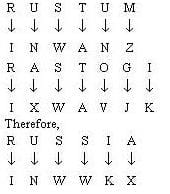RRB JE ME (CBT I) Mock Test- 3 - Question 51

If the 3rd day of a month is Monday, which day will fall on the 5th day after the 21st of that month?

Detailed Solution for RRB JE ME (CBT I) Mock Test- 3 - Question 51 The 3rd day is Monday. So, the 10th and 17th days are also Mondays.

Thus, the 21st day is Friday.

.'. The fifth day from the 21st will be Wednesday.

RRB JE ME (CBT I) Mock Test- 3 - Question 52

Read the following information carefully and answer the questions given below it.

‘X + Y’ means ‘X is the father of Y’.

‘X – Y’ means ‘X is the mother of Y’

‘X x Y’ means ‘X is the brother of Y’

‘X ÷ Y’ means ‘X is the sister of Y.’

If L + M x N÷P – R then L is R’s?

Detailed Solution for RRB JE ME (CBT I) Mock Test- 3 - Question 52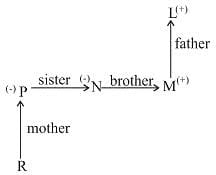L is the father of P who is the mother of R. Thus, L is the maternal grandfather of R.

RRB JE ME (CBT I) Mock Test- 3 - Question 53

Find the odd one out of, the given alternatives.

Detailed Solution for RRB JE ME (CBT I) Mock Test- 3 - Question 53 The second number is one less than the square of the first number.
RRB JE ME (CBT I) Mock Test- 3 - Question 54

Find the odd figure/word/number pair from the given responses.

Detailed Solution for RRB JE ME (CBT I) Mock Test- 3 - Question 54 The second number is one more than the cube of the first number
RRB JE ME (CBT I) Mock Test- 3 - Question 55

Arrange the following in a logical order:

1. Butterfly

2. Cocoon

3. Egg

4. Worn

Detailed Solution for RRB JE ME (CBT I) Mock Test- 3 - Question 55 The logical arrangement of the occurrence of various steps in a process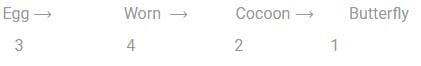RRB JE ME (CBT I) Mock Test- 3 - Question 56

Which is at the apex of the three-tier system of Panchayati Raj?

Detailed Solution for RRB JE ME (CBT I) Mock Test- 3 - Question 56 Zila Parishad is at the apex of the three-tier system of Panchayati Raj.
RRB JE ME (CBT I) Mock Test- 3 - Question 57

How many volumes of the history of Akbar’s reign were written by Abul Fazl?

Detailed Solution for RRB JE ME (CBT I) Mock Test- 3 - Question 57
• The Akbarnama which translates to Book of Akbar, is the official chronicle of the reign of Akbar, the third Mughal Emperor (r. 1556–1605), commissioned by Akbar himself by his court historian and biographer, Abul Fazl .

• The Akbarnama is a document of history of Akbar's reign and his ancestors spread over three volumes.

• It contains the history of Akbar's ancestors from Timur to Humayun, Akbar's reign up to the 46th regnal year (1602), and an administrative report of Akbar's empire, the Ain-i-Akbari, which itself is in three volumes.

RRB JE ME (CBT I) Mock Test- 3 - Question 58

Shimla Agreement 1972 between India & Pakistan was signed in

Detailed Solution for RRB JE ME (CBT I) Mock Test- 3 - Question 58 The Simla Agreement (or Shimla Agreement) was signed between India and Pakistan on 2nd July 1972 in Barnes court (Raj Bhavan), Simla, the capital city of the Indian state of Himachal Pradesh. The agreement sought to lay down the principles that should govern their future relations. It also envisaged steps to be taken for further normalization of bilateral relations. Most importantly, it bounded the two countries "to settle their differences by peaceful means through bilateral negotiations".
RRB JE ME (CBT I) Mock Test- 3 - Question 59

In which battle against Mysore the East India Company ultimately won a victory?

Detailed Solution for RRB JE ME (CBT I) Mock Test- 3 - Question 59
• The fourth and final war was fought between the British and Mysore in 1799, in which Seringapatam was taken, and Tipu was killed in its defence.

• This was the final conflict of the four Anglo–Mysore Wars. The British captured the capital of Mysore.

• In the fourth war, the East India Company ultimately won.

RRB JE ME (CBT I) Mock Test- 3 - Question 60

The famous Sanchi Stupa was commissioned by-

Detailed Solution for RRB JE ME (CBT I) Mock Test- 3 - Question 60 The famous Sanchi Stupa was commissioned by ASHOKA.The Great Stupa at Sanchi is one of the oldest stone structures in India and an important monument of Indian Architecture. It was originally commissioned by the emperor Ashoka in the 2nd century BCE. It is a simple hemispherical brick structure built over the relics of the Buddha.
RRB JE ME (CBT I) Mock Test- 3 - Question 61

Which among the following choices can cause a Tsunami (also known as harbour wave)?

Detailed Solution for RRB JE ME (CBT I) Mock Test- 3 - Question 61 A tsunami is a series of large waves generated by an abrupt movement on the ocean floor that can result from an earthquake, an underwater landslide, a volcanic eruption or very rarely a large meteorite strike.
RRB JE ME (CBT I) Mock Test- 3 - Question 62

In which year the Sisodiya Capital of Chittor was seized by Akbar?

Detailed Solution for RRB JE ME (CBT I) Mock Test- 3 - Question 62
• Chittorgarh also known as chittor is home to the Chittor Fort.

• The Chittor Fort is said to have been built by Chitrangada, a king of the local Maurya dynasty (not to be confused with the imperial Mauryans).

• In 1535, Bahadur Shah of Gujarat besieged and conquered the fort. After he was driven away by the Mughal emperor Humayun, the Sisodias regained control of the fort.

• In 1567-68, the Mughal emperor Akbar besieged and captured the fort.

RRB JE ME (CBT I) Mock Test- 3 - Question 63

In the Republic of India, the administrative head of a Union Territory is -

Detailed Solution for RRB JE ME (CBT I) Mock Test- 3 - Question 63 A union territory is a type of administrative division in the Republic of India. Unlike the states of India, which have their own governments, union territories are federal territories ruled directly by the union government (central government), hence the name "union territory" and are governed by Lieutenant Governor.
RRB JE ME (CBT I) Mock Test- 3 - Question 64

Heat and light in the Sun is produced by the process of__________?

Detailed Solution for RRB JE ME (CBT I) Mock Test- 3 - Question 64 The Sun is about halfway through its main-sequence stage, during which nuclear fusion reactions in its core fuse hydrogen into helium. Each second, more than four million tons of matter are converted into energy within the Sun's core, producing neutrinos and solar radiation.
RRB JE ME (CBT I) Mock Test- 3 - Question 65

State Chief Minister plays the role of Vice chairman in which of the following organization?

Detailed Solution for RRB JE ME (CBT I) Mock Test- 3 - Question 65 Zonal council is divided into five parts i.e. east, west, north, south and central. The Union Home Minister is the Chairman of each of these Councils. The Chief Ministers of the States included in each zone act as Vice-Chairman of the Zonal Council for that zone by rotation, each holding office for a period of one year at a time.
RRB JE ME (CBT I) Mock Test- 3 - Question 66

Which section of Indian Constitution has often been referred to as its 'conscience'?

Detailed Solution for RRB JE ME (CBT I) Mock Test- 3 - Question 66
• Fundamental rights are often regarded as the conscience of the Indian constitution as it provides due guarantee for the protection of rights and regulations of the citizens.

• It is even regarded as the keystone of the democracy through which entire functioning in the nation takes place under set of defined rules.

RRB JE ME (CBT I) Mock Test- 3 - Question 67

Prior to independence, the first Indian jute mill was set up in __________.

Detailed Solution for RRB JE ME (CBT I) Mock Test- 3 - Question 67 Prior to independence, Seth Hukumchand, a Marwari businessman set up the first ever jute mill in Bengal.
RRB JE ME (CBT I) Mock Test- 3 - Question 68

Where can you find the Kunchikal waterfalls?

Detailed Solution for RRB JE ME (CBT I) Mock Test- 3 - Question 68 Kunchikal Falls is located in Nidagodu village near Masthikatte in Shimoga district of state Karnataka. Kunchikal falls cascades down rocky boulders and the total height of fall is 455 meters according to world waterfall database. Kunchikal falls is formed by Varahi river.
RRB JE ME (CBT I) Mock Test- 3 - Question 69

Which of the following is called 'The queen of Arabian sea'?

Detailed Solution for RRB JE ME (CBT I) Mock Test- 3 - Question 69 Kochi is the Queen of Arabian Sea, tucked in the beauty of coconut palms and endless blue waters, is one of the important towns in south India, with a natural harbour. It is the commercial hub of Kerala. Kochi is a dynamic city with soaring land prices.
RRB JE ME (CBT I) Mock Test- 3 - Question 70

In which state is the World Heritage Site 'Basilica of Bom Jesus' located?

Detailed Solution for RRB JE ME (CBT I) Mock Test- 3 - Question 70 Churches and convents of Old Goa is the name given by UNESCO to a set of religious monuments located in Goa Velha (or Old Goa), in the state of Goa, India, which were declared a World Heritage Site in 1986. Goa was the capital of Portuguese India and Asia and an evangelization center from the sixteenth century.
RRB JE ME (CBT I) Mock Test- 3 - Question 71

Which one of the following is true about the center of gravity if a body is in unstable equilibrium?

Detailed Solution for RRB JE ME (CBT I) Mock Test- 3 - Question 71 The center of gravity should be as high as possible. The center of gravity is a geometric property of any object the center of gravity is the average location of the weight of an object.
RRB JE ME (CBT I) Mock Test- 3 - Question 72

How many Newton’s Laws of Motion are there?

Detailed Solution for RRB JE ME (CBT I) Mock Test- 3 - Question 72 There are 3 Newton’s Laws of Motion
RRB JE ME (CBT I) Mock Test- 3 - Question 73

Which of the following will be displaced by the other three in its salt solution?

Detailed Solution for RRB JE ME (CBT I) Mock Test- 3 - Question 73 Less reactive metal will be displaced by more reactive metal in its salt solution.
RRB JE ME (CBT I) Mock Test- 3 - Question 74

How many periods are there in the Periodic Table?

Detailed Solution for RRB JE ME (CBT I) Mock Test- 3 - Question 74 There are 7 Periods in the Periodic Table.
RRB JE ME (CBT I) Mock Test- 3 - Question 75

For instant energy, athletes take:

Detailed Solution for RRB JE ME (CBT I) Mock Test- 3 - Question 75 Glucose readily mixes in the blood and gives energy, thus it is the go-to for athleteThe digestive system breaks down carbohydrates in foods and drinks into simple sugars, mainly glucose. For example, both rice and soft drink will be broken down to simple sugars in your digestive system. This simple sugar is then carried to each cell through the bloodstream.

The pancreas secretes a hormone called insulin, which helps the glucose to migrate from the blood into the cells. Once inside a cell, the glucose is ‘burned’ along with oxygen to produce energy. Our brain, muscles and nervous system all rely on glucose as their main fuel to make energy.

The body converts excess glucose from food into glycogen. Glycogen acts as a storage form of glucose within the muscle tissue and the liver. Its role is to supplement blood sugar levels if they drop between meals or during physical activity.

RRB JE ME (CBT I) Mock Test- 3 - Question 76

Which of the following is an Ideal Fluid?

Detailed Solution for RRB JE ME (CBT I) Mock Test- 3 - Question 76 A fluid with zero viscosity is called an Ideal Fluid. It doesn’t exist in reality .
RRB JE ME (CBT I) Mock Test- 3 - Question 77

Longest cell in human body is

Detailed Solution for RRB JE ME (CBT I) Mock Test- 3 - Question 77 Nerve cell (Neuron) is the longest cell in human body, with a length of 90-100 cm. Its jointed the Central Nervous system to other parts of the body.
RRB JE ME (CBT I) Mock Test- 3 - Question 78

Woolen clothes keep us warm in winter because:

Detailed Solution for RRB JE ME (CBT I) Mock Test- 3 - Question 78 Cotton clothes are thin and do not have space in which air can be trapped. Thus, cotton clothes do not prevent heat coming out of our body. Woollen clothes keep us warm during winter because wool is a poor conductor of heat and it has air trapped in between the fibres.
RRB JE ME (CBT I) Mock Test- 3 - Question 79

Solids which conduct electricity at higher temperature but not at lower temperature are called

Detailed Solution for RRB JE ME (CBT I) Mock Test- 3 - Question 79 Semiconductors are insulators at low temperatures and reasonably good conductors at higher temperatures. As temperature increases, the Semiconductor material becomes a better and better conductor.
RRB JE ME (CBT I) Mock Test- 3 - Question 80

For the reversibility of a cycle, there should be

Detailed Solution for RRB JE ME (CBT I) Mock Test- 3 - Question 80 For the reversibility of a cycle, there should be no loss of energy
RRB JE ME (CBT I) Mock Test- 3 - Question 81

The minimum velocity which must be attained by a rocket to leave the earth’s atmosphere is known as escape velocity. Its value is

Detailed Solution for RRB JE ME (CBT I) Mock Test- 3 - Question 81 11.2 km/s-The minimum velocity which must be attained by a rocket to leave the earth’s atmosphere is known as escape velocity and its value is 11.2 km/s.
RRB JE ME (CBT I) Mock Test- 3 - Question 82

Which of the following is an example of inertia?

Detailed Solution for RRB JE ME (CBT I) Mock Test- 3 - Question 82 Both of the above are examples of inertia
RRB JE ME (CBT I) Mock Test- 3 - Question 83

Communication satellites are used to:

Detailed Solution for RRB JE ME (CBT I) Mock Test- 3 - Question 83 A communication satellite is basically a self-contained communications system with the ability to receive signals from Earth and to retransmit those signals back with the use of a transponder-an integrated receiver and transmitter of radio signals.
RRB JE ME (CBT I) Mock Test- 3 - Question 84

A sudden fall in the level of mercury in a barometer indicates.

Detailed Solution for RRB JE ME (CBT I) Mock Test- 3 - Question 84 stormy weather- A sudden fall in the mercury level in a barometer indicates stormy weather.
RRB JE ME (CBT I) Mock Test- 3 - Question 85

A family consumes 40 kg. of fuel in 20 days. Calculate the average energy consumed per day (calorific value of fuel = 40 KJ/g).

Detailed Solution for RRB JE ME (CBT I) Mock Test- 3 - Question 85 Mass of fuel consumed in

20 days = 40 kg. = 40000g.

Energy liberated by 1 g. of fuel

= 40 KJ.

Energy liberated by 40000 g. of fuel = 40 × 40000 KJ Average energy consumption

= (40 x 40000)/20 = 80000 K J

= 8 x 104 KJ

RRB JE ME (CBT I) Mock Test- 3 - Question 86

Which of the following rays exhibits the property of light wave?

Detailed Solution for RRB JE ME (CBT I) Mock Test- 3 - Question 86 Gamma rays, X-rays, microwaves and radio waves behave as a light wave and they exhibit properties such as reflection, refraction, diffraction etc.
RRB JE ME (CBT I) Mock Test- 3 - Question 87

Surface tension in a liquid is due to ___________.

Detailed Solution for RRB JE ME (CBT I) Mock Test- 3 - Question 87 Surface tension is a contractive tendency of the surface of a liquid that allows it to resist an external force. This property is caused by cohesion of similar molecules, and is responsible for many of the behaviors of liquids. It is revealed, for example, in the floating of some objects on the surface of water, even though they are denser than water, and in the ability of some insects (e.g. water striders) to run on the water surface.
RRB JE ME (CBT I) Mock Test- 3 - Question 88

A ball thrown vertically upwards with an initial velocity of 19.6 m/s returns in 4 seconds. Which one of the following is the maximum height attained by the ball?

Detailed Solution for RRB JE ME (CBT I) Mock Test- 3 - Question 88 Time taken by ball to the Highest point = 4/2

= 2 Second

Now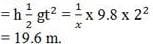RRB JE ME (CBT I) Mock Test- 3 - Question 89

How many chromosomes pairs are in a human body?

Detailed Solution for RRB JE ME (CBT I) Mock Test- 3 - Question 89 In humans, each cell normally contains 23 pairs of chromosomes, for a total of 46. Twenty-two of these pairs, called autosomes, look the same in both males and females. The 23rd pair, the sex chromosomes, differ between males and females

A chromosome is a deoxyribonucleic acid (DNA) molecule with part or all of the genetic material (genome) of an organism. Most eukaryotic chromosomes include packaging proteins which, aided by chaperone proteins, bind to and condense the DNA molecule to prevent it from becoming an unmanageable tangle.

Chromosomes are normally visible under a light microscope only when the cell is undergoing the metaphase of cell division (where all chromosomes are aligned in the center of the cell in their condensed form). Before this happens, every chromosome is copied once (S phase), and the copy is joined to the original by a centromere, resulting either in an X-shaped structure (pictured to the right) if the centromere is located in the middle of the chromosome or a two-arm structure if the centromere is located near one of the ends. The original chromosome and the copy are now called sister chromatids. During metaphase the X-shape structure is called a metaphase chromosome. In this highly condensed form chromosomes are easiest to distinguish and study. In animal cells, chromosomes reach their highest compaction level in anaphase during chromosome segregation.

Chromosomal recombination during meiosis and subsequent sexual reproduction play a significant role in genetic diversity. If these structures are manipulated incorrectly, through processes known as chromosomal instability and translocation, the cell may undergo mitotic catastrophe. Usually, this will make the cell initiate apoptosis leading to its own death, but sometimes mutations in the cell hamper this process and thus cause progression of cancer.

RRB JE ME (CBT I) Mock Test- 3 - Question 90

A transformer works with

Detailed Solution for RRB JE ME (CBT I) Mock Test- 3 - Question 90 A transformer works with Alternating current.
RRB JE ME (CBT I) Mock Test- 3 - Question 91

Which of the following is chemical change?

Detailed Solution for RRB JE ME (CBT I) Mock Test- 3 - Question 91 Wood burning in a fireplace Chemical change is any change that results in the formation of new chemical substances. At the molecular level, chemical change involves making or breaking of bonds between atoms. These changes are chemical: iron rusting (iron oxide forms)
RRB JE ME (CBT I) Mock Test- 3 - Question 92

Which law states that at a constant temperature, the volume of a definite mass of a gas is inversely proportional to pressure?

Detailed Solution for RRB JE ME (CBT I) Mock Test- 3 - Question 92 Boyle’s Law states that at a constant temperature, the volume of a definite mass of a gas is inversely proportional to pressure
RRB JE ME (CBT I) Mock Test- 3 - Question 93

The material having maximum ductility is:

Detailed Solution for RRB JE ME (CBT I) Mock Test- 3 - Question 93 The material having maximum ductility is Mild steel
RRB JE ME (CBT I) Mock Test- 3 - Question 94

The smallest living organisms with cell wall are-

Detailed Solution for RRB JE ME (CBT I) Mock Test- 3 - Question 94 Bacteria are the smallest single-celled prokaryotes with cell walls. Cyanobacteria (blue-green algae), yeast and algae are all larger than bacteria.
RRB JE ME (CBT I) Mock Test- 3 - Question 95

Which of the following applications don’t use the Bernoulli’s principal?

Detailed Solution for RRB JE ME (CBT I) Mock Test- 3 - Question 95 Boat floating on water can be described by Archimedes principal.
RRB JE ME (CBT I) Mock Test- 3 - Question 96

Which of the following is called the ‘Kitchen of the Cell’?

Detailed Solution for RRB JE ME (CBT I) Mock Test- 3 - Question 96 The Chloroplast is called the Kitchen of the Cell as it is the green pigment found in green plants involved in the photosynthesis.
RRB JE ME (CBT I) Mock Test- 3 - Question 97

The SI unit of impulse is -

Detailed Solution for RRB JE ME (CBT I) Mock Test- 3 - Question 97 It is Newton Seconds, Ns
RRB JE ME (CBT I) Mock Test- 3 - Question 98

When 1 mol of a gas is heated at constant volume, temperature is raised from 298 to 308 K. Heat supplied to the gas is 500 J. Then which statement is correct?

Detailed Solution for RRB JE ME (CBT I) Mock Test- 3 - Question 98 At constant volumes, dV = 0. Hence W = 0. Further

dU = q + w As W = 0, dU = q = 500 J

RRB JE ME (CBT I) Mock Test- 3 - Question 99

Magnetic effect of current was discovered by

Detailed Solution for RRB JE ME (CBT I) Mock Test- 3 - Question 99 Magnetic effect of current was discovered by Oersted.
RRB JE ME (CBT I) Mock Test- 3 - Question 100

When gold is melted and formed in a mold to make a piece of jewelry, what type of change is taking place?

Detailed Solution for RRB JE ME (CBT I) Mock Test- 3 - Question 100 Physical change- When gold is melted and formed in a mold to make a piece of jewelry. It is a physical change.

## RRB JE Mock Test Series for Mechanical Engineering (ME)

158 tests
 Use Code STAYHOME200 and get INR 200 additional OFF Use Coupon Code
Information about RRB JE ME (CBT I) Mock Test- 3 Page
In this test you can find the Exam questions for RRB JE ME (CBT I) Mock Test- 3 solved & explained in the simplest way possible. Besides giving Questions and answers for RRB JE ME (CBT I) Mock Test- 3 , EduRev gives you an ample number of Online tests for practice

158 tests